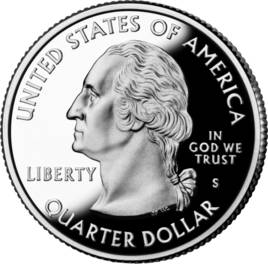# 数学文化，一元钱、两元钱，为啥没有三元钱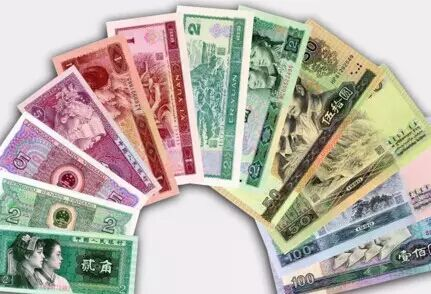1=1　　（1张）
2=2　　（1张）
3=1+2　（2张）
4=2+2　（2张）
5=5　　（1张）
6=1+5　（2张）
7=2+5　（2张）
8=1+2+5（3张）
9=2+2+5（3张）

1=1　　（1张）
2=2　　（1张）
3=3　　（1张）
4=2+2　（2张）
5=5　　（1张）
6=1+5　（2张）
7=2+5　（2张）
8=3+5　（2张）
9=2+2+5（3张）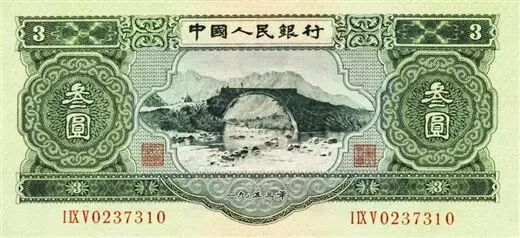“苏三币”虽然短命，但实际上我国货币面额使用3这个数字有着悠久的历史。西汉武帝时就铸造过名曰“三铢”的铜钱。这恐怕是世界上最早以3作为面额的货币了。以后历代都出现过“3”、“30”、“300”等面额的各种货币。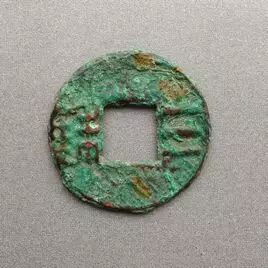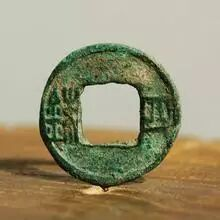6也没闲着。篡汉的王莽建立新朝，铸造的布货十品中的“中布六百”，可能是世界上最早以6为面额的货币。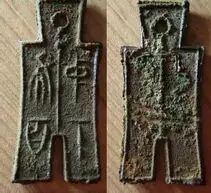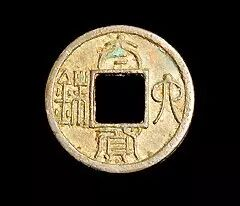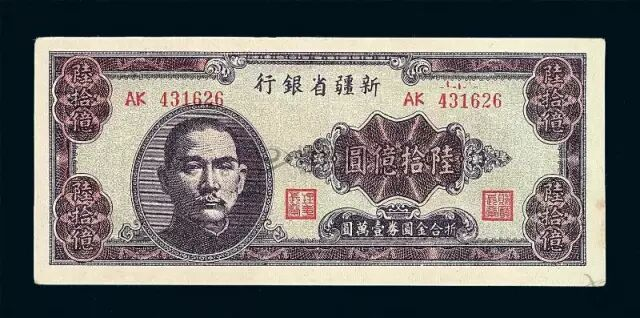7、8出现在钱币上极为罕见，而9在我国历史上冠以这个数字的钱币更是仅有一种，即王莽布货十品中的“次布九百”布币。